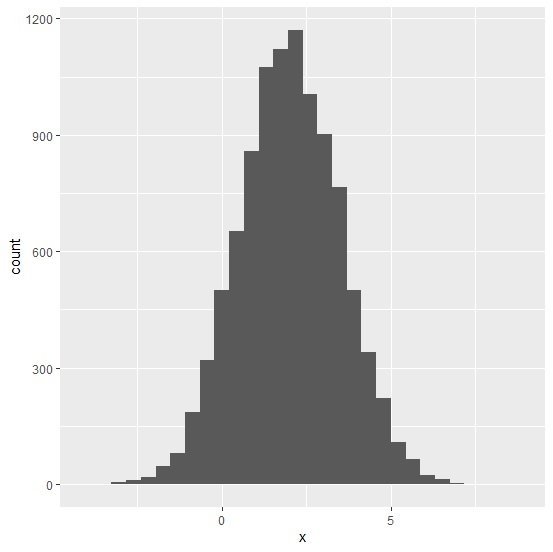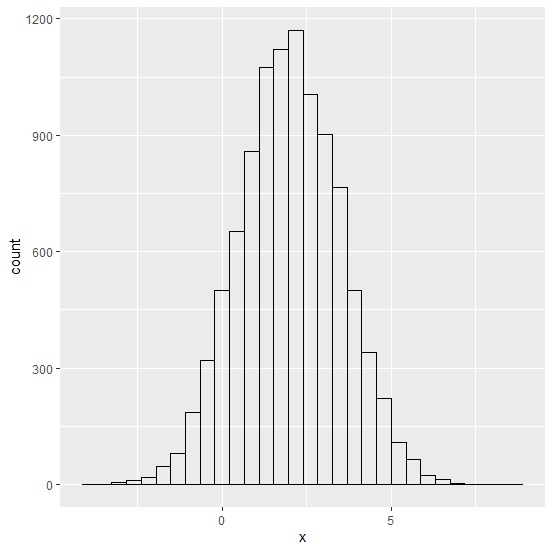# How to create a transparent histogram using ggplot2 in R?

When we create a histogram using ggplot2 package, the area covered by the histogram is filled with grey color but we can remove that color to make the histogram look transparent. This can be done by using fill="transparent" and color="black" arguments in geom_histogram, we need to use color argument because if we don’t use then the borders of the histogram bars will also be removed and this color is not restricted to black color only.

## Example

Consider the below data frame −

set.seed(987)
x<-rnorm(10000,2,1.5)
df<-data.frame(x)

library(ggplot2) ggplot(df,aes(x))+geom_histogram(bins=30)

## OutputCreating the transparent histogram −

ggplot(df,aes(x))+geom_histogram(bins=30,fill="transparent",color="black")

## Output